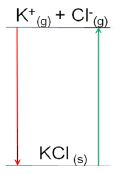Chemistry

# Describe on Lattice Enthalpy

The strength of the covalent bonds can be measured by the bond enthalpy. In ionic compounds the comparable enthalpy value which relates to the strength of the ionic bonds is called the lattice enthalpy.

Lattice enthalpy is a measure of the attraction of all of the positive ions to all the surrounding negative ions in the crystal structure.

Definition: lattice enthalpy ΔH° is the enthalpy change when one mole of an ionic crystal is formed from the ions in a gaseous state under standard conditions.

Always look carefully at the equation or definition given in a question to see which is being used.

K+ (g) + CI (g) → KCI (s) ΔHOL < 0

KCl (s) → K+ (g)+ Cl (g) ΔHOL ˃ 0

The above reactions can be drawn as shown in the Figure below: